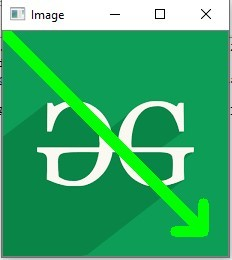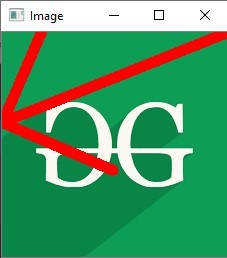# Python OpenCV | cv2.arrowedLine() method

OpenCV-Python is a library of Python bindings designed to solve computer vision problems.
`cv2.arrowedLine()` method is used to draw arrow segment pointing from the start point to the end point.

Syntax: cv2.arrowedLine(image, start_point, end_point, color[, thickness[, line_type[, shift[, tipLength]]]])

Parameters:
image: It is the image on which line is to be drawn.
start_point: It is the starting coordinates of line. The coordinates are represented as tuples of two values i.e. (X coordinate value, Y coordinate value).
end_point: It is the ending coordinates of line. The coordinates are represented as tuples of two values i.e. (X coordinate value, Y coordinate value).
color: It is the color of line to be drawn. For BGR, we pass a tuple. eg: (255, 0, 0) for blue color.
thickness: It is the thickness of the line in px.
line_type: It denotes the type of the line for drawing.
shift: It denotes number of fractional bits in the point coordinates.
tipLength: It denotes the length of the arrow tip in relation to the arrow length.
Return Value: It returns an image.

Image used for all the below examples:Example #1:

 `# Python program to explain cv2.arrowedLine() method  ` ` `  `# importing cv2  ` `import` `cv2  ` ` `  `# path  ` `path ``=` `r``'C:\Users\Atomix\Desktop\geeksforgeeks\geeks.png'` ` `  `# Reading an image in default mode  ` `image ``=` `cv2.imread(path)  ` ` `  `# Window name in which image is displayed  ` `window_name ``=` `'Image'` ` `  `# Start coordinate, here (0, 0)  ` `# represents the top left corner of image  ` `start_point ``=` `(``0``, ``0``)  ` ` `  `# End coordinate ` `end_point ``=` `(``200``, ``200``)  ` ` `  `# Green color in BGR  ` `color ``=` `(``0``, ``255``, ``0``)  ` ` `  `# Line thickness of 9 px  ` `thickness ``=` `9` ` `  `# Using cv2.arrowedLine() method  ` `# Draw a diagonal green arrow line ` `# with thickness of 9 px  ` `image ``=` `cv2.arrowedLine(image, start_point, end_point, ` `                                     ``color, thickness)  ` ` `  `# Displaying the image  ` `cv2.imshow(window_name, image)  `

Output:Example #2:

 `# Python program to explain cv2.arrowedLine() method  ` ` `  `# importing cv2  ` `import` `cv2  ` ` `  `# path  ` `path ``=` `r``'C:\Users\Atomix\Desktop\geeksforgeeks\geeks.png'` ` `  `# Reading an image in default mode  ` `image ``=` `cv2.imread(path)  ` ` `  `# Window name in which image is displayed  ` `window_name ``=` `'Image'` ` `  `# Start coordinate, here (225, 0)  ` `# represents the top right corner of image  ` `start_point ``=` `(``225``, ``0``)  ` ` `  `# End coordinate ` `end_point ``=` `(``0``, ``90``)  ` ` `  `# Red color in BGR  ` `color ``=` `(``0``, ``0``, ``255``)  ` ` `  `# Line thickness of 9 px  ` `thickness ``=` `9` ` `  `# Using cv2.arrowedLine() method  ` `# Draw a red arrow line ` `# with thickness of 9 px and tipLength = 0.5 ` `image ``=` `cv2.arrowedLine(image, start_point, end_point,  ` `                    ``color, thickness, tipLength ``=` `0.5``)  ` ` `  `# Displaying the image  ` `cv2.imshow(window_name, image)  `

Output:Attention geek! Strengthen your foundations with the Python Programming Foundation Course and learn the basics.

To begin with, your interview preparations Enhance your Data Structures concepts with the Python DS Course.

My Personal Notes arrow_drop_upCheck out this Author's contributed articles.

If you like GeeksforGeeks and would like to contribute, you can also write an article using contribute.geeksforgeeks.org or mail your article to contribute@geeksforgeeks.org. See your article appearing on the GeeksforGeeks main page and help other Geeks.

Please Improve this article if you find anything incorrect by clicking on the "Improve Article" button below.

Article Tags :

Be the First to upvote.

Please write to us at contribute@geeksforgeeks.org to report any issue with the above content.